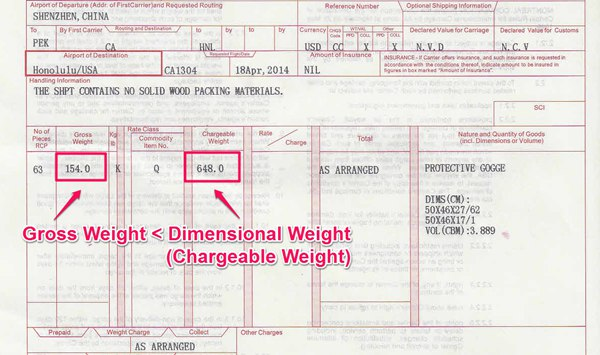info@chinatsfreight.com

China +86 185 7568 6246

USA +1 (310) 707 1816

# How To Calculate Air Chargeable Weight When Importing From China

Calculation of chargeable weight for air freight shipping when importing goods from China; is quite simple. A standard method of calculation that is applied universally, is used. Charges are computed on the basis of the cargo weight. Though, what confuses most exporters and importers is whether they need to pay freight charges based on actual weight or dimensional weight? The rule of thumb is that air freight is always calculated based on the higher of the two.

Understanding Air Chargeable Weight

What is chargeable weight and how is it worked out? For calculating chargeable weight the actual weight of the cargo and its volume are both considered. The significance of chargeable weight and why it is used in air freight shipping can be better understood through the following example –

All commodities do not have the same volume. Some may weigh less but have a larger volume. Take for instance 1 ton of steel as compared to 1 ton of cotton. The latter will occupy more space than the former. Here, the volume of cotton is much higher than that of steel, even though it weighs the same and will require more space on the carrier.

If the airfreight charges are levied according to weight of the cargo alone; both packages will be charged the same, irrespective of the fact that the cotton cargo is inadvertently occupying a lot more space. If you consider this factor you will understand the significance of chargeable weight and why it becomes important to consider actual weight and volume of cargo.

How Is Air Chargeable Weight Calculated

For calculating dimensional weight the exact dimensions of the cargo packages are required. That is the length x breadth x height.

• If the measurements are taken in centimeters, then the formula for calculation will be (length x breadth x height) cm3 divided by 6,000.
• If the measurements are in meters then the formula that is applied for calculation will be length x breadth x height CBM (cubic meters) divided by 0.006.

Air carriers use above calculations to determine whether to charge air freight based on gross weight or dimensional weight. Remember, the freight rate is based on which of the two is higher, which we call chargeable weight.

This can be better explained through actual examples. Here are measurements and weight of two shipments and the calculation for air freight –

Example I

Gross Weight: 154kgs
Dimensions of cargo (cm): 50 x 46x 27 = 62 cartons 50 x 46 x 17 = 1 carton
Cubic Size Calculation: 50 x 46 x 27 x 62 + 50 x 46 x 17 x 1 = 3889300 cubic centimeter
Dimensional Weight Calculation: 3889300/6000 = 648kgs

In this example the gross weight of the shipment is 154kgs and the dimensional weight is 648kgs. Since the dimensional weight is greater than the gross weight of the cargo the air freight will be levied for the dimensional weight, 648kgs becomes the chargeable weight.Example II

Gross Weight: 400lbs
Dimensions of cargo: 12″(Length) X12″ (Width)X 10″(Height)
Packages: 100
Cubic Size Calculation: 12 x 12 x 10 x 100 =144,000 cubic inches
Dimensional Weight Calculation: 144,000/166 = 867lbs
Chargeable Weight: 867lbs

Irrespective of which system is used for calculation of volume and weight the net results are always the same. It does not make a difference whether you are using kgs and lbs for calculating weight and inches/meters/centimeters for volume.